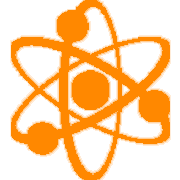#### SCI-MS.PS2.016th Grade (SCI) Physical Science Standards
[PS2] Motion and Stability: Forces and Interactions

##### SCI-MS.PS2.01 Apply Newton's Third Law to design a solution to a problem involving the motion of two colliding objects.

Clarification Statement: Emphasis is on balanced (Newton’s First Law) and unbalanced forces in a system, qualitative comparisons of forces, mass and changes in motion (Newton’s Second Law), frame of reference, and specification of units.
Disciplinary Core Ideas
PS2.A: Forces and Motion The motion of an object is determined by the sum of the forces acting on it; if the total force on the object is not zero, its motion will change. The greater the mass of the object, the greater the force needed to achieve the same change in motion. For any given object, a larger force causes a larger change in motion.

## Student Learning Targets:

### Knowledge Targets

• I can describe Newton's Third Law of Motion.
• I can identify action and reaction force pairs.

• I can

### Skills (Performance) Targets

• I can find the momentum of an object given its mass and velocity.

### Product Targets

• I can construct different types of models to represent different systems and their interactions.

## Proficiency Scale

##### 1 Beginning
... with help, demonstrate a partial understanding of some of the simpler details and processes (Score 2.0 content) and some of the more complex ideas and processes (Score 3.0 content).
• descriptors
##### 2 Developing
... demonstrate no major errors or omissions regarding the simpler details and processes but exhibits major errors or omissions regarding the more complex ideas and processes (Score 3.0 content).
• Key Vocabulary: Newton's Third Law; input/output, energy, motion, matter, force, momentum, inertia, action, reaction, mass, velocity
• can calculate momentum of an object given its mass and velocity
##### 3 Proficient
“The Standard.”
... demonstrate no major errors or omissions regarding any of the information and processes that were end of instruction expectations.
• apply Newton's Third Law of Motion
• identify and explain for every action there is an opposite and equal reaction. (Third Law)
Sample Activity:
Rube Goldberg Machine
Roller Coaster Lab
Connect each law to a situation

... demonstrate in-depth inferences and applications regarding more complex material that go beyond end of instruction expectations.
• descriptors

## Resources

### Vocabulary

• Newton's 3rd Law of Motion
• energy
• motion
• matter
• force
• inertia
• Action/ Reaction Forces
• Momentum, Mass, Velocity

### Websites

• Title of website with a URL to open in a new window